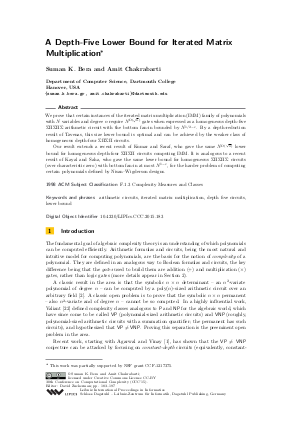Document# A Depth-Five Lower Bound for Iterated Matrix Multiplication

### Authors Suman K. Bera, Amit Chakrabarti## File

LIPIcs.CCC.2015.183.pdf
• Filesize: 476 kB
• 15 pages

## Cite As

Suman K. Bera and Amit Chakrabarti. A Depth-Five Lower Bound for Iterated Matrix Multiplication. In 30th Conference on Computational Complexity (CCC 2015). Leibniz International Proceedings in Informatics (LIPIcs), Volume 33, pp. 183-197, Schloss Dagstuhl - Leibniz-Zentrum für Informatik (2015)
https://doi.org/10.4230/LIPIcs.CCC.2015.183

## Abstract

We prove that certain instances of the iterated matrix multiplication (IMM) family of polynomials with N variables and degree n require N^(Omega(sqrt(n))) gates when expressed as a homogeneous depth-five Sigma Pi Sigma Pi Sigma arithmetic circuit with the bottom fan-in bounded by N^(1/2-epsilon). By a depth-reduction result of Tavenas, this size lower bound is optimal and can be achieved by the weaker class of homogeneous depth-four Sigma Pi Sigma Pi circuits. Our result extends a recent result of Kumar and Saraf, who gave the same N^(Omega(sqrt(n))) lower bound for homogeneous depth-four Sigma Pi Sigma Pi circuits computing IMM. It is analogous to a recent result of Kayal and Saha, who gave the same lower bound for homogeneous Sigma Pi Sigma Pi Sigma circuits (over characteristic zero) with bottom fan-in at most N^(1-epsilon), for the harder problem of computing certain polynomials defined by Nisan-Wigderson designs.
##### Keywords
• arithmetic circuits
• iterated matrix multiplication
• depth five circuits
• lower bound

## Metrics

• Access Statistics
• Total Accesses (updated on a weekly basis)
0